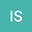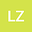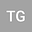Stream-Aligned Magnetohydrodynamics for Solar Wind Simulations
•••Lulu Zhao
University of Michigan, University of Michigan
Author Profile## Abstract

We present a reduced magnetohydrodynamic (MHD) mathematical model describing the dynamical behavior of highly conducting plasmas with frozen-in magnetic fields, constrained by the assumption that, there exists a frame of reference, where the magnetic field vector, B, is aligned with the plasma velocity vector, u, at each point. We call this solution “stream-aligned MHD” (SA-MHD). Within the framework of this model, the electric field, E = −u x B ≡ 0, in the induction equation vanishes identically and so does the electromagnetic energy flux (Poynting flux), E x B ≡ 0, in the energy equation. At the same time, the force effect from the magnetic field on the plasma motion (the Ampere force) is fully taken into account in the momentum equation. Any steady-state solution of the proposed model is a legitimate solution of the full MHD system of equations. However, the converse statement is not true: in an arbitrary steady-state magnetic field the electric field does not have to vanish identically (its curl has to, though). Specifically, realistic tree-dimensional solutions for the steady-state (ambient) solar atmosphere in the form of so-called Parker (1958) spirals, can be efficiently generated within the stream-aligned MHD (SA-MHD) with no loss in generality.
01 Feb 2022Published in The Astrophysical Journal volume 926 issue 1 on pages 102. 10.3847/1538-4357/ac400f